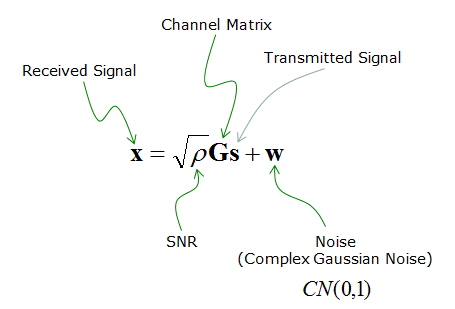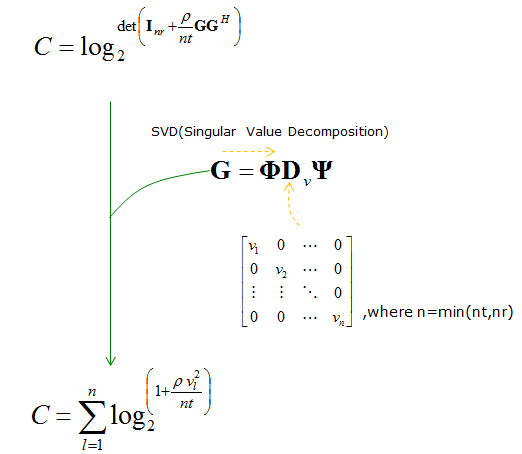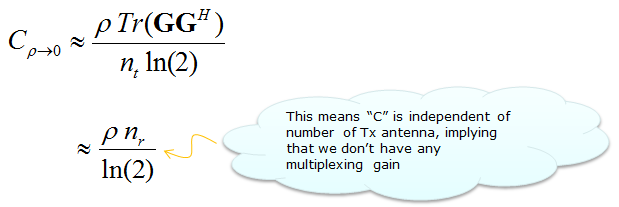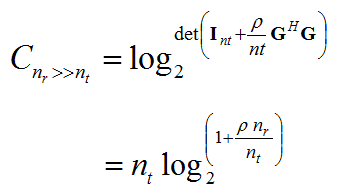5G - Massive MIMO                                                          Home : www.sharetechnote.com Massive MIMO Channel Model - Examples   Following is based on Scaling up MIMO : Opportunities and Challenges with Very Large Arrays   Channel Model : Following is the brief mathematicl description of channel model for MIMOAchievable Rate : Following is the mathematical model expressing the achievable data rate. This is generic form and you see all the factors (e.g, Number of Tx, Rx Antenna, SNR, Channel Matrix) in the equation. But depending on the situation, the dominant factors gets different and you can approximate this generic for to various other (simpler) form.Another way of expressing this formular is as follows. This equation represents the same thing using the diagonal numbers of Singular Matrix obtained by SVD. (SVD is pretty important mathematical tools for MIMO modeling. If you are not familiar with this, see SVD page for understanding the concept and refer to LTE MIMO page to see an application)When SNR is very low as in cell edge, this equation can be approximated as follows. (How the orginal equation can  be approximated like this is described  in detail in this paper. You may need to look for another paper if you are really understand the details.)When the number of transmitter antenna gets very large comparing to the number of reciever antenna, following term can be approximated to an Identity Matrix. (How this approximation can be derived is not described in this paper)Using this approximation, you can rewrite the original equation as shown below.When the number of reciever antenna gets very large comparing to the number of transmitter antenna, the original equation can be expressed as follows.Reference    Scaling up MIMO : Opportunities and Challenges with Very Large Arrays Fredrik Rusek, Dept. of Electrical and Information Technology, Lund University, Lund, Sweden Daniel Persson, Dept. of Electrical Engineering (ISY), Linkoping University, Sweden Buon Kiong Lau, Dept. of Electrical and Information Technology, Lund University, Lund, Sweden Erik G. Larsson, Dept. of Electrical Engineering (ISY), Linkoping University, Sweden Thomas L. Marzetta, Bell Laboratories, Alcatel-Lucent, Murray Hill, NJ Ove Edfors, Dept. of Electrical and Information Technology, Lund University, Lund, Sweden Fredrik Tufvesson, Dept. of Electrical and Information Technology, Lund University, Lund, Sweden